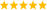# LabVIEW

cancel
Showing results for
Did you mean:

## Re: Same code gives different results in Matlab Script in Labview and Matlab

Thanks.. Here's the matlab code and the vi is attached. When I make changes, I start getting errors like matrix dimension exceeded. error 1050, 1047. I restart matlab and it starts to work but same strategy doesn't apply everytime. Can someone plz tell me what's going on inside which stops the program?

Matlab

S=400;
n_eff_re=linspace(0.5,1.5,S);
n_eff_im=linspace(0.06,0.15,S);

c=299792458;
a=0.1e-6;
b=0.1e-6;
L=a+b;
na=1;
n=[2.89 3.38];
lambda = 1.15e-6;
omega = 2*pi*c/lambda;
beta_re=n_eff_re.*omega/c;
beta_im=n_eff_im.*omega/c;
W=zeros(S);
N=10;
for i = 1:S
for j=1:S
beta_c = beta_re(i) + 1i*beta_im(j);

k = [sqrt((omega*n(1)/c)^2 - (beta_c^2))  sqrt((omega*n(2)/c)^2 - (beta_c^2))];
A = exp(-1i*k(1)*a)* ((cos(k(2)*b) - (1i/2)*(k(2)/k(1) + k(1)/k(2))*sin(k(2)*b)));
D = conj(A);
B = exp(1i*k(1)*a)*((-1i/2)*(k(2)/k(1) - k(1)/k(2))*sin(k(2)*b));
C = conj(B);
K = (1/L)*(acos((A+D)/2));
q_a = sqrt(beta_c^2 - (omega*na/c)^2);
q = sqrt(beta_c^2 - (omega*n(1)/c)^2);
W(i,j)=abs((A+((q_a - q)/(q_a +q))*C)*(sin(N*K*L)/sin(K*L))-((sin((N-1)*K*L))/(sin(K*L))));
end
end
contourf(n_eff_re,n_eff_im,W)

Message 11 of 22
(456 Views)

## Re: Same code gives different results in Matlab Script in Labview and Matlab

Hi kikki,

I would recommend checking out these KnowledgeBase Articles for common reasons for these errors:

Why Do I Get Error 1047 When Trying to Use The MATLAB Script Node in LabVIEW?

Error 1050 Occurred in MATLAB Script Node: Complex Values Cannot be Converted to Chars.

Like altenbach and RavensFan suggested, have you tried transposing the array to see if this resolves the issue?

Allison M.
Applications Engineer
National Instruments
ni.com/support
Message 12 of 22
(435 Views)

## Re: Same code gives different results in Matlab Script in Labview and Matlab

Hello!

I know this thread is old, but it seems am experiencing some similar problems. But even simpler.

I have some recorded data, at 100Hz, and I load them and compute the derivative. In Matlab is pretty smooth (I am executing with only disctrete time samples and so on, and when I zoom I can see the stairs each 0.01s, so is fine), but when the same is done in Labview I can see some short of "undersampling", which does not occur all time, and therefore the derivative goes crazy. I attach the graph cmparison for 10sec. Top is matlab (yellow uploaded siganl and magenta de derivative) and down is labview (white uploaded signal and red derivative).Message 13 of 22
(351 Views)

## Re: Same code gives different results in Matlab Script in Labview and Matlab

We probably would need to see some code. Make sure to use exactly equivalent functions in both scenarios.LabVIEW Champion. It all comes together inGCentralWhat does "Engineering Redefined" mean??
Message 14 of 22
(339 Views)

## Re: Same code gives different results in Matlab Script in Labview and Matlab

Hello, thank you for your reply. I actually use the same code, because I am usig formula Node in Labview and a script in matlab. Basically what it does is:

TS=-RawData*pi/180;
TS=(TS-TS_Ant)/T;
TS=(T*TS+0.02*TS_Ant)/(0.02+T);

So is extracting one elemnt of a big vector and computing the filtered derivative. Actually RawData is a big matrix with 4000samples of a 11 element vector. So I use a foor loop to extract sequentially each item

Message 15 of 22
(327 Views)

## Re: Same code gives different results in Matlab Script in Labview and Matlab

How hard would it be to attach actual code?LabVIEW Champion. It all comes together inGCentralWhat does "Engineering Redefined" mean??
Message 16 of 22
(322 Views)

## Re: Same code gives different results in Matlab Script in Labview and Matlab

Sorry, I did not want to fill everything with uploads. Here I attache the Labview program I am using to figure out what is wrong

I think maybe is something it does when uploading the RawData into de model...I cannot upload the RawData, is too big 😞

Message 17 of 22
(315 Views)

## Re: Same code gives different results in Matlab Script in Labview and Matlab

Did you adjust for the fact that Matlab array indexes start at 1 while LV array indexes start at 0?

Lynn

Message 18 of 22
(302 Views)

## Re: Same code gives different results in Matlab Script in Labview and Matlab

Yeap, I took special care with that

Message 19 of 22
(298 Views)

## Re: Same code gives different results in Matlab Script in Labview and Matlab

can you attach a small input file that has only 2 or 3 rows?

Can you tell us what the matlab output is for this data?

Can you also show us the matlab code?

(Some quick glances: I would eliminate the local variable "T" and wire directly to thet terminal. Now you can even delete the big sequence structure. Why is "TS_Ant" initilized with an array of size 155? You never seem to go that high. The first element of the TS array written to the output file will always be zero, is that intentional?)LabVIEW Champion. It all comes together inGCentralWhat does "Engineering Redefined" mean??
Message 20 of 22
(289 Views)